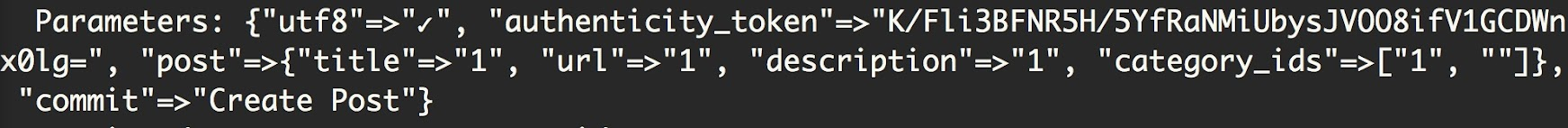# Rails - 拆解 Form Helper 以 Checkbox 為例

## Collection Check Box

``````<%= f.label "Categories" %>
<%= f.collection_check_boxes :category_ids, Category.all, :id, :name do |cb| %>
<%= cb.label( class:"checkbox inline"){cb.check_box(class:"checkbox")+cb.text} %>
<% end %>
``````

``````<input id="post_category_ids_1" name="post[category_ids][]" type="checkbox" value="1">
<label for="post_category_ids_1">food</label>
<input id="post_category_ids_2" name="post[category_ids][]" type="checkbox" value="2">
<label for="post_category_ids_2">Play</label><input name="post[category_ids][]" type="hidden" value="">
``````## 手刻html版的checkbox

``````    <% Category.all.each do |category| %>
<input id="post_category_ids_<%= category.id %>" name="post[category_ids][<%= category.id %>]"
type="checkbox" value="<%= category.id %>" />
<label for="post_category_ids_<%= category.id %>"><%= category.name %></label>
<% end  %>
``````

Tags:

Updated: